# Math- Universal Themes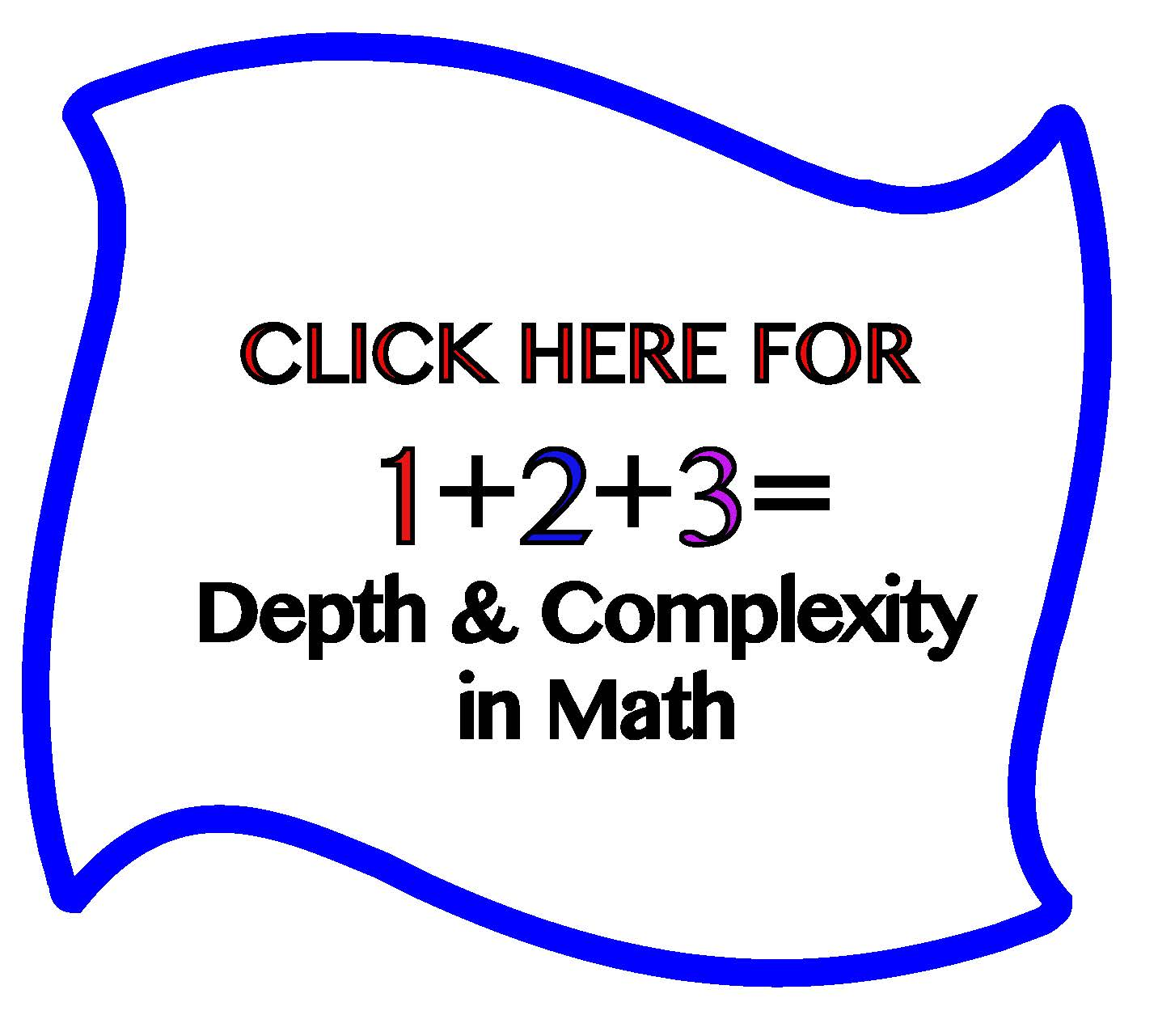Use universal themes in mathematics!  Because universal themes are universal, they can be applied across content

areas, and mathematics is no exception.  This approach is one way to differentiate instruction for gifted students and those who have high capacity in mathematics.

## Universal Themes in Math- Structure• Prime/composite numbers
• Factors
• Fractions/ Decimals
• Geometry/ Measurement
• Area and Perimeter
• Symmetry
• Coordinate Grid
• Graphs/ Data Analysis

Have students prove universal themes in math.  In the example to the right, students used the generalization, “Relationships serve a purpose“, to explore integers.  In this lesson, students explored the parallels between the images to better understand how they were related.  After charting the relationships, I introduced the big idea for the lesson- Integers.  Having this understanding at the start of the lesson helped students to have a more conceptual understanding of the topic.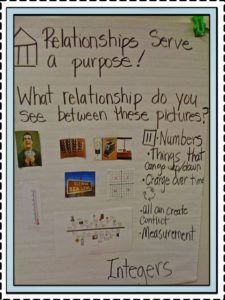## Using the Universal Theme, “Order” in the Elementary ClassroomLooking for ways to integrate the universal theme, order into your math classroom?  You can use the generalization, “Order has a purpose” to help students make a connection to order and their own learning in mathematics.
Take for example, the role order has in understanding place value.  By understanding the order (tens, hundreds, etc) you can more easily understand the value to which each are applied.

## Universal Themes and Iconic Statements in Math

Having students prove iconic statements (universal truths) requires a very high level of thinking.

## Rules Help us Determine Missing Details!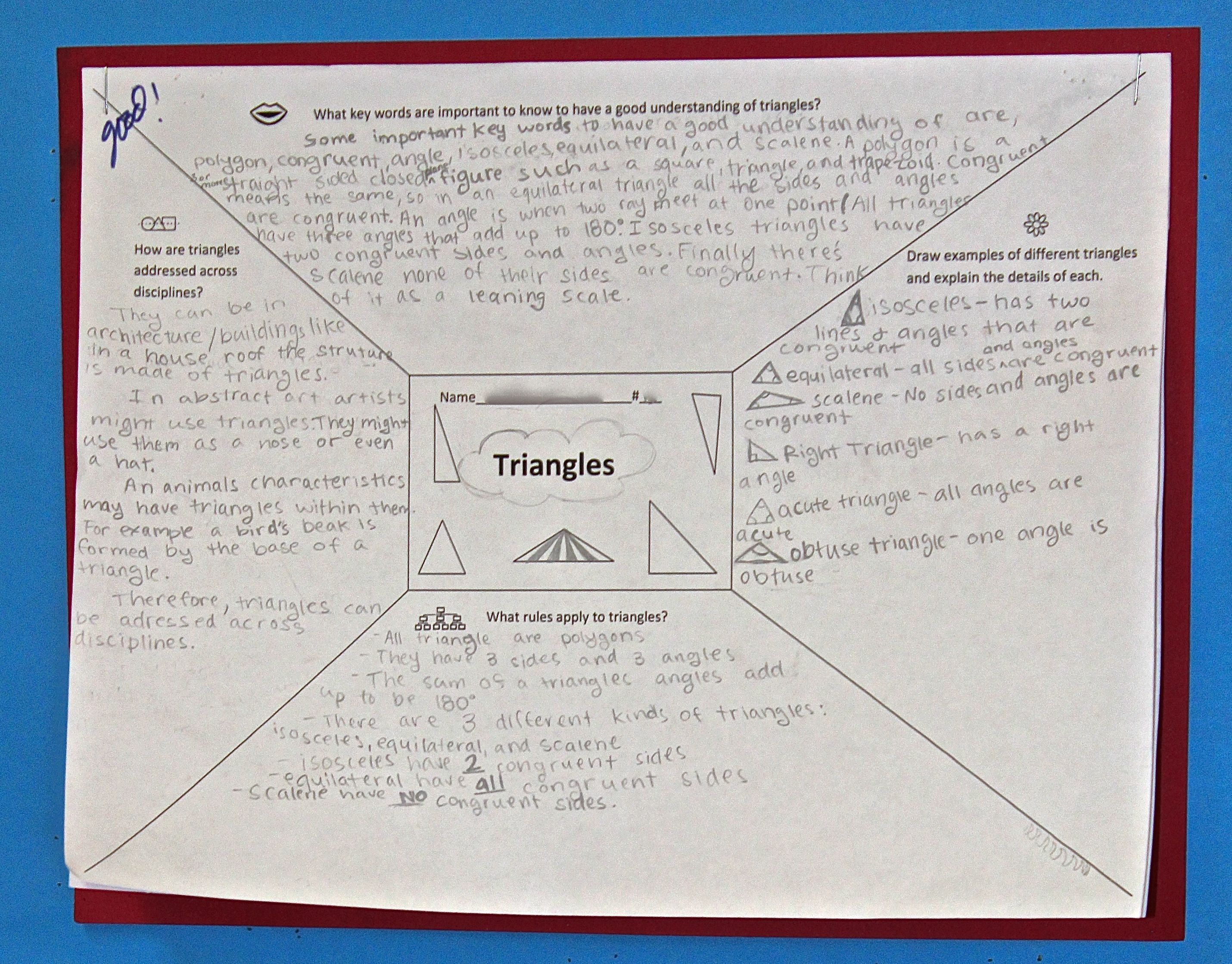Students demonstrated understanding of mathematical concepts while proving the premise, “understanding rules helps to determine missing details”.

## Examining Details Allows us to See Patterns and Relationships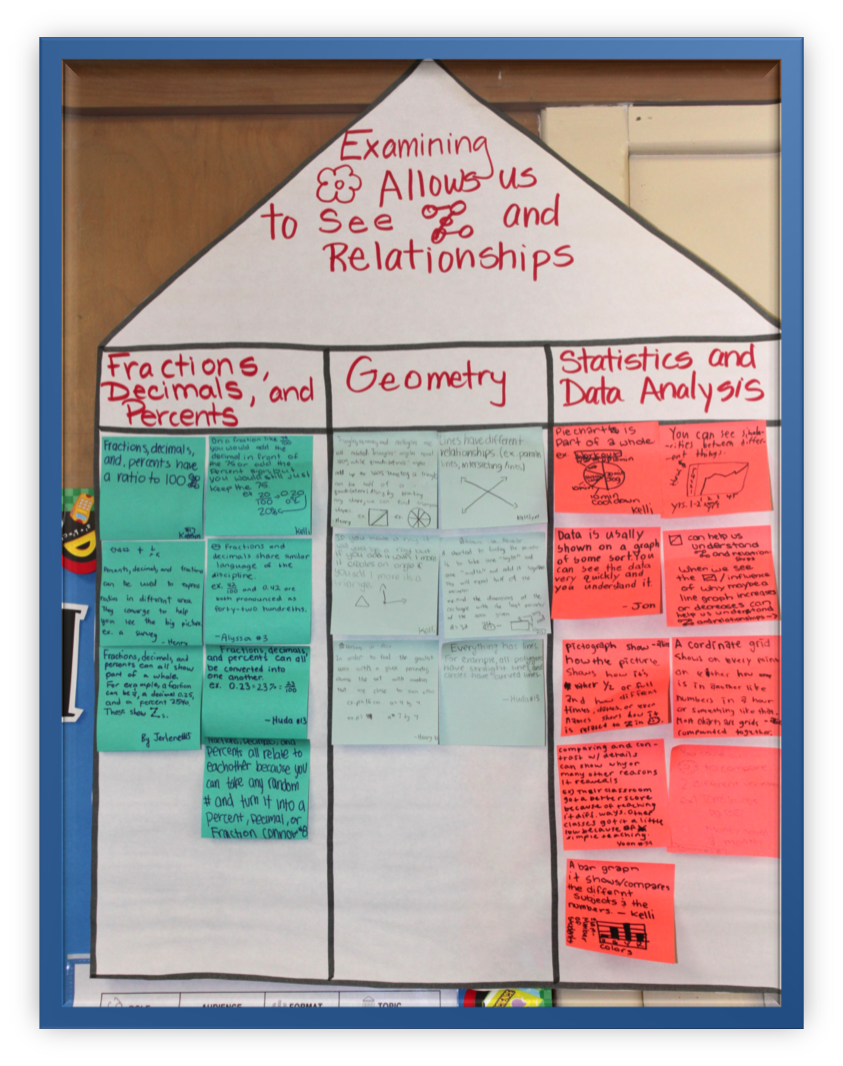In this example, students were pressed to support the premise: examining details allows us to see patterns and relationships in math.  This was done as a culminating activity toward the end of the year.

## Rules are Revealed Through Patterns!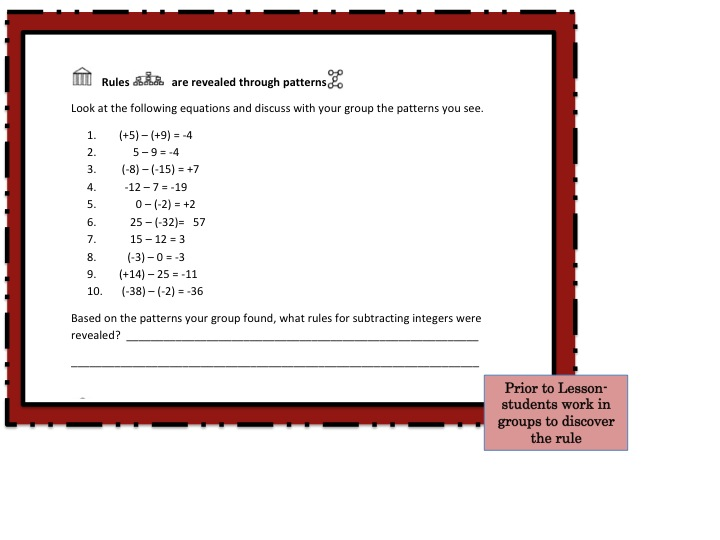Students worked in groups prior to the lesson to note details and patterns that emerged from examining the document at the left.  Armed with this information, they discovered the mathematical rule that applied to the concept we would be learning.  Students applied the rule to problems presented during the lesson.

SaveSave